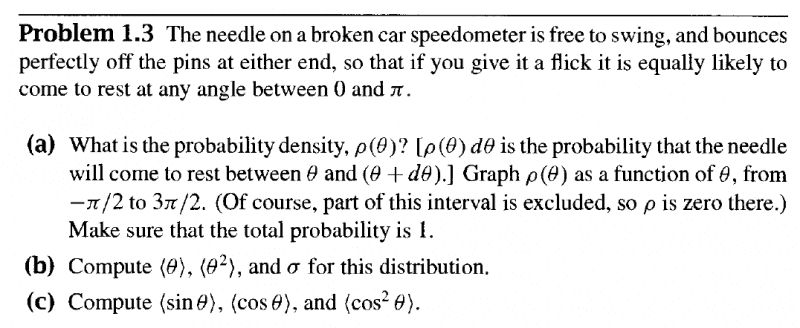# Solving for probability density (Griffith's 1.3)

• gfd43tg
In summary, the conversation discusses the concept of probability density and its relation to wave functions in the context of a physics problem. The question is posed about how to determine the wave function in the given scenario, and the solution is to simply assume that the probability density is constant, leading to a final conclusion that the probability density is equal to 1/pi. The conversation also touches upon the basic understanding of probability and its connection to wave functions in quantum mechanics.

Gold Member

## Homework Statement

Problem statement in image## The Attempt at a Solution

From my reading, I know that the probability density
$$\langle x \rangle = \int x |\psi|^{2} \hspace 0.05 in dx$$

Where ##\psi## is the wave function and ##\langle x \rangle## is the probability density. So I figure that I will go ahead and do this,

$$\rho (\theta) = \int \theta |\psi|^{2} \hspace 0.05 in d \theta$$

However, how do I figure out the wave function of this? I don't have a good sense for this problem and can't connect the pieces.

#### Attachments

Last edited:
What you have written down is the expectation value, not the probability density. It is stated in the problem what the probability density is and what properties it should have.

Okay, so then I interpret the question to mean when it says that if flicked, it is equally likely to come to rest at any angle between zero and pi

$$P = \int_{0}^{\pi} \rho (\theta) \hspace 0.02 in d \theta = 1$$

So if I assume ##\rho (\theta)## is constant, then ##\rho (\theta) = \frac {1}{\pi}##

Correct. It does not have to be harder than that :)

Maylis said:
Okay, so then I interpret the question to mean when it says that if flicked, it is equally likely to come to rest at any angle between zero and pi

$$P = \int_{0}^{\pi} \rho (\theta) \hspace 0.02 in d \theta = 1$$

So if I assume ##\rho (\theta)## is constant, then ##\rho (\theta) = \frac {1}{\pi}##

No assumption needed; that is exactly what is meant by "equally likely".

You seem to have some basic misunderstandings of probability. It usually has nothing to do with Quantum Mechanics or wave functions and the like. If we talk about probabilities in coin-tossing, there are no wave functions involved. If we talk about outcomes when spinning a roulette wheel, no wave functions are involved. When we talk about probabilities of car accidents, no wave functions are involved. When I want to know how long I have to wait for a bus, no wave functions come into the issue. Only when we talk about probabilities of events at the atomic or subatomic scale do wave functions enter into the calculation.